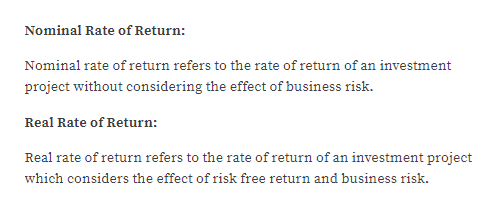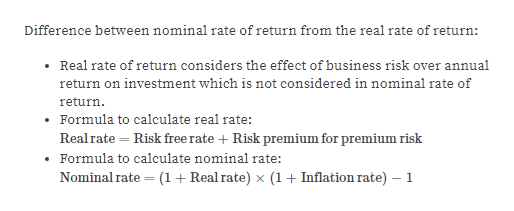# Distinguish the nominal rate of return from the real rate of return.

Question
1 views

Distinguish the nominal rate of return from the real rate of return.

check_circle

Step 1help_outlineImage TranscriptioncloseNominal Rate of Return: Nominal rate of return refers to the rate of return of an investment project without considering the effect of business risk. Real Rate of Return: Real rate of return refers to the rate of return of an investment project which considers the effect of risk free return and business risk. fullscreen
Step 2help_outlineImage TranscriptioncloseDifference between nominal rate of return from the real rate of return: • Real rate of return considers the effect of business risk over annual return on investment which is not considered in nominal rate of return. Formula to calculate real rate: Real rate = Risk free rate + Risk premium for premium risk Formula to calculate nominal rate: (1+ Real rate) x (1+ Inflation rate) Nominal rate fullscreen

### Want to see the full answer?

See Solution

#### Want to see this answer and more?

Solutions are written by subject experts who are available 24/7. Questions are typically answered within 1 hour.*

See Solution
*Response times may vary by subject and question.
Tagged in